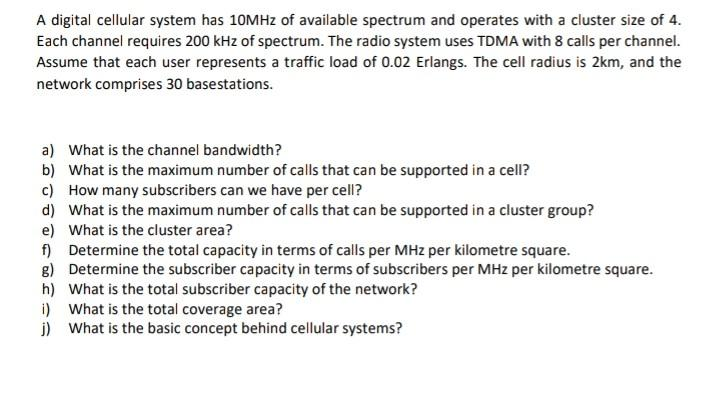Home / Expert Answers / Electrical Engineering / q2-2-cellular-planning-a-digital-cellular-system-has-10mhz-of-available-spectrum-and-operates-with-pa262

# (Solved): Q2.2 Cellular planning A digital cellular system has 10MHz of available spectrum and operates with ...

Q2.2 Cellular planning
A digital cellular system has 10MHz of available spectrum and operates with a cluster size of 4.
Each channel requires 200 kHz of spectrum. The radio system uses TDMA with 8 calls per channel.
Assume that each user represents a traffic load of 0.02 Erlangs. The cell radius is 2km, and the
network comprises 30 basestations.
a) What is the channel bandwidth?
b) What is the maximum number of calls that can be supported in a cell?
c) How many subscribers can we have per cell?d) What is the maximum number of calls that can be supported in a cluster group?
e) What is the cluster area?
f) Determine the total capacity in terms of calls per MHz per kilometre square.
g) Determine the subscriber capacity in terms of subscribers per MHz per kilometre square.
h) What is the total subscriber capacity of the network?
i) What is the total coverage area?
j) What is the basic concept behind cellular systems?

A digital cellular system has of available spectrum and operates with a cluster size of 4 . Each channel requires of spectrum. The radio system uses TDMA with 8 calls per channel. Assume that each user represents a traffic load of 0.02 Erlangs. The cell radius is , and the network comprises 30 basestations. a) What is the channel bandwidth? b) What is the maximum number of calls that can be supported in a cell? c) How many subscribers can we have per cell? d) What is the maximum number of calls that can be supported in a cluster group? e) What is the cluster area? f) Determine the total capacity in terms of calls per per kilometre square. g) Determine the subscriber capacity in terms of subscribers per per kilometre square. h) What is the total subscriber capacity of the network? i) What is the total coverage area? j) What is the basic concept behind cellular systems?

We have an Answer from Expert